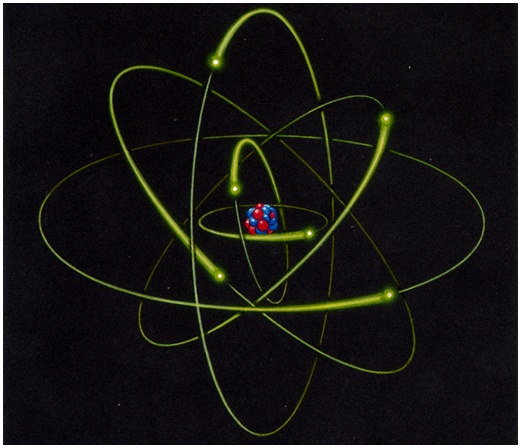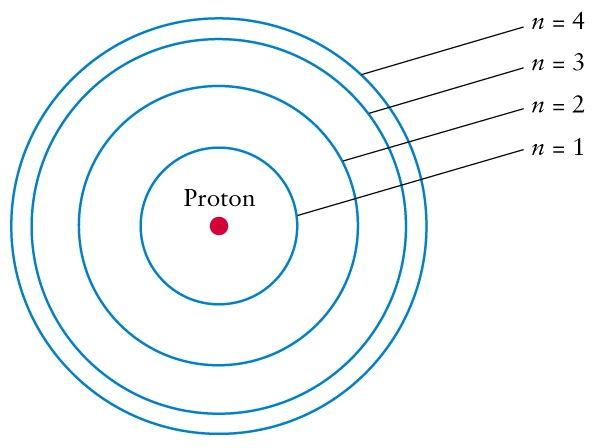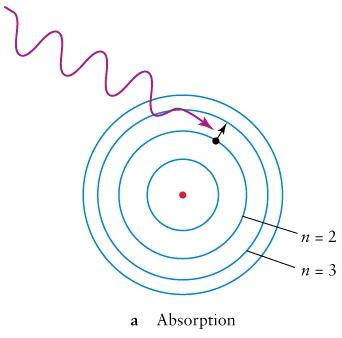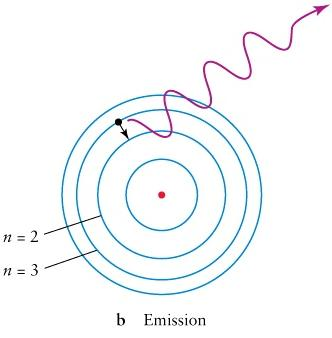# Lecture 5: Discrete Spectra of Atoms

Chapter 5: 8th Ed. pages 108 - 123 or 3rd Ed. pages 108 - 123

## Structure of an Atom

• A typical atom has a diameter close to 10-10 m.
• Most of the mass of an atom is concentrated in a tiny nucleus which has a diameter close to 10-14 m.
• The nucleus is composed of two types of particles: protons and neutrons.
• Electrons move in the region outside of the nucleus.
• We don't know exactly where an electron is, but we can describe the probability that the electron is in a certain region called an orbital.Next

## Quantum Mechanics and Atoms

### Bohr Model of Hydrogen

• Electrons can only "orbit" the atom's nucleus at special locations.
• Each special location corresponds to a particular amount of energy called an energy level.
• Energy levels are discrete . We enumerate them with integers n .
• Bohr's model assumes that the orbitals are circular orbits.
• This is not what really happens, because orbital is determined by two quantities -- energy and angular momentum of the electron.
• But Bohr's model is still a useful model if we are interested only in energy• The lowest energy state is called the "ground state" . It corresponds to innermost n=1 orbit on the diagram.
• If the electron is in the n=1 energy level, then we say that the atom is in its ground state.
• If the electron is in a higher energy level, n > 1, then we call the atom "excited" .
• E1 is the energy of the ground state, En is the energy of the nth energy level,     En > E1.

Back Next

## Transitions between energy levels

• In order for an atom to become excited, it must absorb enough energy to allow the electron to make a quantum jump to a higher energy level.
• for the electron to make the jump from the ground level to level n, the atom must be supplied with Energy = En - E1.
• There are must be enough energy to excite the atom at least to the next level. Smaller amounts of energy cannot be absorbed nor stored.
• Energy for excitation can come in the form of a photon of light (photo-excitation)
• or it can come from atom collisions (collisional excitation)
• To de-excite, the atom must release energy. Energy is released in discrete amounts.
• Given time, the excited atom will spontaneously de-excite by emitting a photon. It tries to get to the lowest energy state if left to itself !

Back Next

## Absorption of a photon

• Remember, the energy of a photon is Energy = h f where f = frequency and h = Planck's constant = 6.626 x 10-34Js.
• The electron can make the jump from the ground level if the photon has the frequency

f = (En - E1)/h
• If the atom is already excited and is in, say, the N'th level it can jump to the n'th level if the photon has frequency

f = (En - EN)/h
• This process is called absorption since the atom absorbs a photon.
• Only photons with specific frequencies can be absorbed.## Emission of a photon

• The opposite process is called emission
• Emission of a photon occurs when an electron in a high energy state jumps down to a lower energy state.
• If the electron jumps from the n'th energy level to the ground state, a photon emitted with frequency
f = (En - E1)/h
• Frequencies of the emitted photons are discrete.Back Next

## Example: Hydrogen atom

 The chemical element is determined by the number of protons in the nucleus. Number of electrons is equal number of protons when atom is not ionized. Hydrogen H has one proton and one electron orbiting it. Energy levels of Hydrogen atom are given by the formulaelectron-volt: 1 eV = 1.60218 x 10-19 Joules. This is a useful unit for atomic energy measurements

### Energy between two levels of the Hydrogen atom### Wavelength of the photon absorbed or emitted in transition between two levels of the Hydrogen atom,Back Next

## Ionization and recombination

• If atom is supplied with too much energy, an electron can be pulled away completely. This process is called ionization
• What is left when electron is pulled away is ion - an atom with less electrons than protons. Ions are electrically charged.
• Minimal energy that needed to ionize an atom can be found by considering transition to n=infinity level. It is called ionization energy
• Ionization energy for Hydrogen from the ground state is 13.6 eV.
• If the environment is hot, most of the atoms are ionized by collisions between them.
• In the opposite process an ion captures the electron. Such process is called recombination

Back Next lecture: Atomic Spectra
Continuing with Chapter 5